# Right Angle Triangle

Here we will learn about right-angled triangles, including what a right-angled triangle is and how to solve problems involving their sides and their angles.

There are also right angle triangle worksheets based on Edexcel, AQA and OCR exam questions, along with further guidance on where to go next if you’re still stuck.

## What is a right angle triangle?

A right angle triangle is a type of triangle that has one right angle ( 90^{\circ} angle).

A right angle is 90^{\circ} and it is denoted using a small square inside the angle at the vertex, instead of the usual arc.

To solve problems involving right-angled triangles, we need to be able to find the values of other missing angles or side lengths and use them where appropriate.

The longest side of a right-angled triangle is called the hypotenuse. It is the opposite side to the right angle.

Properties of triangles are very important within geometry. They are one of the most common shapes to recognise for angles in parallel lines, circle theorems, interior angles, trigonometry, Pythagoras’ theorem and many more. You therefore must be familiar with their individual properties.

### What is a right angle triangle?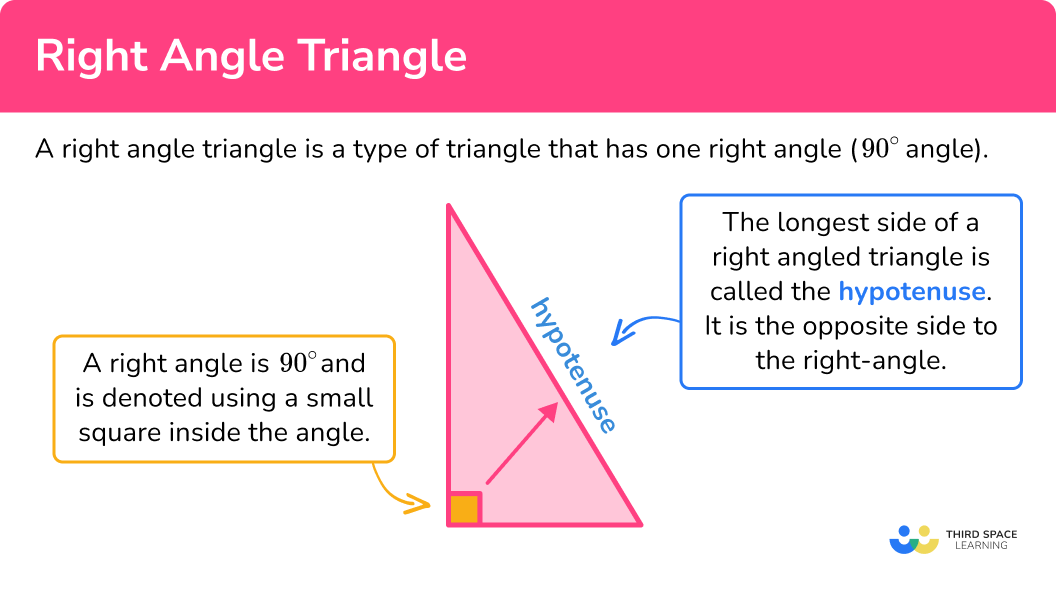### Types of right angle triangles

There are two types of right-angled triangles.

• Right-angled isosceles triangle

A right-angled triangle which also has two equal sides can be known as an isosceles right triangle. This is a special case of a right-angled triangle.

Here, the two equal angles will both be 45^{\circ}.

• Scalene right-angled triangle

A scalene right-angled triangle has all angles of different sizes and all sides of different length. One angle within a scalene right-angled triangle is 90^{\circ}.

### Area of a right angle triangle

The area of a right-angled triangle can be found by using the formula

A=\frac{1}{2}bh

where \text{b} is the base length and \text{h} is the perpendicular height of the triangle. Sometimes these values need to be calculated.

As the triangle contains a right angle, the two sides adjacent to the right angle (not the hypotenuse) are perpendicular to each other, and so these lengths can be used to calculate the triangle’s area.

The area of a triangle is given in square units.

For example, to calculate the area of the right-angled triangle below, we need to know the value for the base \text{b}, and the value of the perpendicular height \text{h}.

Here b=8 and h=6. This is because these two values are perpendicular to each other, despite the base being at “the top” of the image.

The value of 10 \ cm can be a decoy when calculating the area of a triangle as it is not the height of the triangle, it is the hypotenuse, so beware!

Substituting the values for b and h into the area formula, we have

\begin{aligned} A&=\frac{1}{2}bh \\\\ &=\frac{1}{2}\times{8}\times{6} \\\\ &=24\text{ cm}^{2} \end{aligned}

Remember that an area uses square units.

Step-by-step guide: Area of a right angled triangle

### Pythagoras’ theorem

The three sides of a right-angled triangle are linked by a very useful property known as Pythagoras’ theorem.

Pythagoras theorem states that the square of the longest side of a right-angled triangle (called the hypotenuse) is equal to the sum of the squares of the other two sides.

This can be written as

a^2+b^2=c^2.

Side c is always the hypotenuse.

Pythagoas’ theorem can be used to calculate the length of the hypotenuse if we know the other 2 sides and conversely, we can use Pythagoras’ theorem to calculate a shorter side, given the hypotenuse and one other side of a right-angled triangle.

You can find out more about the Pythagorean theorem here.

Step-by-step guide: Pythagoras theorem

### Trigonometry

The sides and angles of right-angled triangles are connected by the trigonometric ratios sine, cosine and tangent. The longest side is still called the hypotenuse.

The shorter sides have names depending on their relationship to the angle, opposite and adjacent (next to).

Step-by-step guide: Trigonometry

## How to solve problems involving right angle triangles

In order to solve problems involving right angle triangles:

1. Locate known angles, including the right angle, and calculate any necessary unknown angles.
2. Locate known sides and calculate any necessary unknown side lengths.
3. Solve the problem using any necessary values.

### Explain how to solve problems involving right angle triangles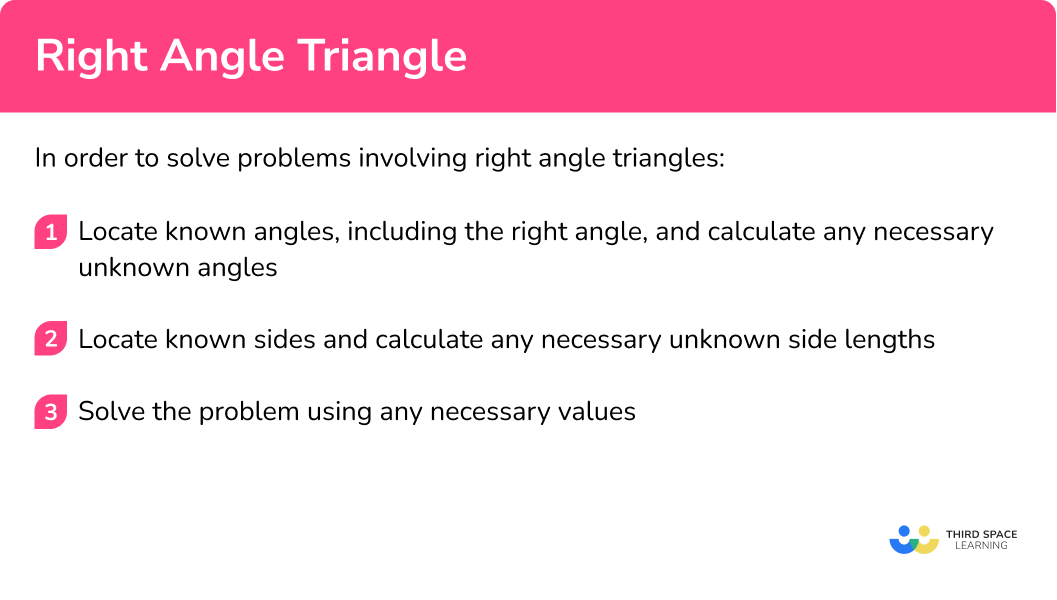### Related lessons on triangles

Right angle triangle is part of our series of lessons to support revision on polygons and triangles. You may find it helpful to start with the main triangles lesson for a summary of what to expect, or use the step by step guides below for further detail on individual topics. Other lessons in this series include:

## Right angle triangle examples

### Example 1: finding the missing angle

Determine the size of angle x.

1. Locate known angles, including the right angle, and calculate any necessary unknown angles.

The triangle contains the three angles, 37^{\circ} (the interior angle at B ), 90^{\circ} (the interior angle at C ), and x^{\circ} (the interior angle at A ). We need to calculate x which we will complete in step 3.

2Locate known sides and calculate any necessary unknown side lengths.

We do not need to know the length of any side to solve this problem.

3Solve the problem using any necessary values.

As the sum of angles in a triangle is 180^{\circ}, we can use this to find the missing angle x by subtracting the other two known angles from 180.

180-(90+37)=53^{\circ}

The angle x=53^{\circ}.

### Example 2: finding the missing angle

Use angle rules to find the size of angle x.

The triangle contains the two angles, 62^{\circ} and 90^{\circ}.

We need to calculate x and so it is useful to calculate the third angle in the triangle as x is on the same point to this angle. As angles in a triangle total 180^{\circ},

180-(90+62)=28^{\circ}.

Labelling this on the diagram, we have

We do not need to know the length of any side to solve this problem.

As vertically opposite angles are equal and x is vertically opposite the angle 28^{\circ}, we can state that x=28^{\circ}.

### Example 3: missing angle

ABC is a right-angled triangle. D lies on the line BC . Determine the size of angle CAD , labelled x.

In the diagram there are 3 known angles, 25^{\circ}, 73^{\circ}, and 90^{\circ}, and 3 unknown angles, one of which is our value of x.

As the angle x is within the triangle ADC , and we know the angle at C \ (25^{\circ}), we need to know the angle at D (angle ADC ). To determine angle ADC , we can see that it is adjacent to the angle ADB and as angles on a straight line total 180^{\circ},

180-73=107^{\circ}.

So angle ADC = 107^{\circ} .

We do not need to calculate any other angle in the triangle for this version of a solution.

We do not need to know the length of any side to solve this problem.

Now, as angles in a triangle total 180^{\circ}, the value of x is equal to

180-(107+25)=180-132=48^{\circ}.

The angle x=48^{\circ}.

Alternative Solution

We need to use the angle fact that the sum of angles in a triangle is 180^{\circ}.

The diagram is made up of two right-angled triangles.

The missing angle on this right angle triangle is

180-(90+25)=65^{\circ} .

The missing angle on this right angle triangle is

180-(90+73)=17^{\circ}.

Angle x will be the difference in these two angles.

65-17=48^{\circ}

Angle x=48^{\circ}

### Example 4: perimeter problem with congruent right angle triangles

Shape ABC is made from two congruent right angle triangles connected at the line BD . The sides of one of the right-angled triangles are 5 \ cm, 12 \ cm and 13 \ cm and AC < BD . Calculate the perimeter of the shape.

We can add the right angles to the diagram. This tells us that the length AC is perpendicular to the width BD . Any further angles in the shape are not needed as we are focused on the perimeter, which is a length.

We are told that the three side lengths of one of the right-angled triangles are 5 \ cm, 12 \ cm, and 13 \ cm.

As the longest side of the triangle is the hypotenuse which is the side opposite the right angle, we can see that AB and BC are both 13 \ cm.

As AC < BD and AC is twice the length of AD, AD must be equal to 5 \ cm as AC would then equal 10 \ cm, and BD would equal 12 \ cm.

Labelling all of these sides, we have

We can now work out the perimeter of the shape.

5+5+13+13=36\text{ cm}

The perimeter of the shape is 36 \ cm.

### Example 5: perimeter of congruent triangles

The shape is made from 2 congruent right-angled triangles. The sides of the triangle are 8 \ cm, 15 \ cm and 17 \ cm. Find the perimeter of the shape.

Remember that there is one right angle. We can add the right angles to the diagram.

Remember that the side opposite the right angle is the longest side. The longest side is the hypotenuse. We can add the sides of the right-angled triangles to the diagram.

The missing side is the difference between the 2 shortest sides 15 \ cm and 8 \ cm.

15-8=7

The perimeter can be found by adding together all the sides of the shape.

17+7+17+8+16=64

Therefore the perimeter is 64 \ cm.

### Example 6: perimeter of a right triangle

The sides of a right-angled triangle are in the ratio 3:4:5. The perimeter is 240 \ cm. Calculate the length of the hypotenuse.

Not needed for this question.

Remember that the side opposite the right angle is the longest side. The longest side is the hypotenuse.

We can divide the perimeter by the sum of the parts of the ratio.

240\div (3+4+5)=20

To find the hypotenuse we need to multiply 20 by 5.

20\times 5=100

Therefore the longest side is 100 \ cm.

Note: 3, \ 4, \ 5 is known as a Pythagorean triple as the sum of the square of the two shorter sides is equal to the square of the third side. This means that the triangle must contain a right angle (3^{2}+4^{2}=5^{2}).

### Common misconceptions

• Angles in polygons

Make sure you know your angle properties. Getting these confused causes quite a few misconceptions.

◌ Angles in a triangle total 180^{\circ} .

◌ Angles in a quadrilateral total 360^{\circ} .

• Angle facts

Make sure you know your angle properties.

◌ Adjacent angles on a straight line add up to 180^{\circ} .

◌ Vertically opposite angles are equal.

◌ Alternate angles are equal.

◌ Corresponding angles are equal.

### Practice right angle triangle questions

1. Find the missing angle in the triangle.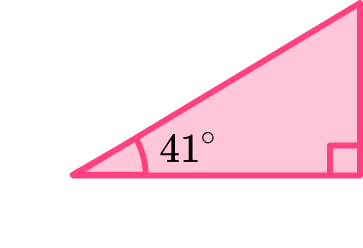39^{\circ}90^{\circ}40^{\circ}49^{\circ}The sum of angles in a triangle is 180^{\circ}. We can use this fact along with the fact that a right angle is 90^{\circ} to find the missing angle.

180-(90+41)=49^{\circ}

The missing angle is 49^{\circ}.

2. Calculate the value of y.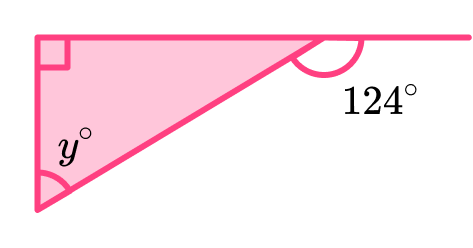56^{\circ}34^{\circ}45^{\circ}65^{\circ}We can use the fact that adjacent angles on a straight line add up to 180^{\circ}.

180-124=56^{\circ}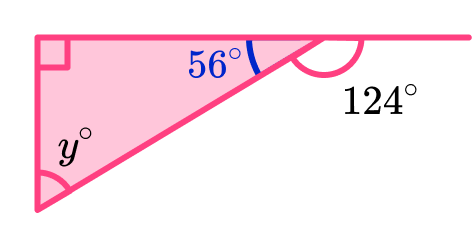As a right angle is 90^{\circ} and angles in a triangle sum to 180^{\circ},

180-(90+56)=34^{\circ}.

The value of y=34^{\circ}.

3. Calculate the size of the smallest angle.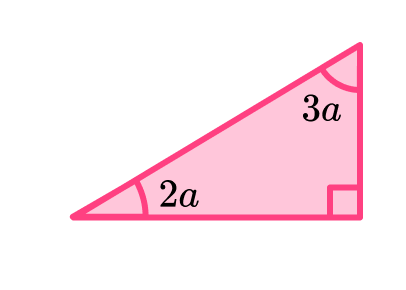45^{\circ}18^{\circ}36^{\circ}54^{\circ}We can use the fact that angles in a triangle add up to 180^{\circ}. This means that the two smaller angles will sum to 90^{\circ} (as 180-90=90^{\circ} ).

\begin{aligned} 2a+3a &=90 \\\\ 5a &= 90 \end{aligned}

Dividing 90 by 5 will determine the value of a.

a=90\div{5}=18

To find the size of the smallest angle, we can multiply 18 by 2.

2\times 18=36

The smallest angle is 36^{\circ}.

4. The sides of a right angle triangle are in the ratio 3:4:5. The perimeter is 96 \ cm. Calculate the length of the shortest side.

40 \ cm24 \ cm8 \ cm32 \ cmWe can divide the perimeter by the sum of the parts of the ratio.

96\div (3+4+5)=8

We can then multiply the 8 by 3 to find the shortest side of the right-angled triangle.

3\times 8=24

The shortest side will be 24 \ cm.

5. The sides of a right angle triangle are 5 \ cm, 12 \ cm, and 13 \ cm. Four of these triangles are put together to make the chevron ABCDEF  (a concave hexagon) where EF < BD.  Calculate the perimeter of the shape.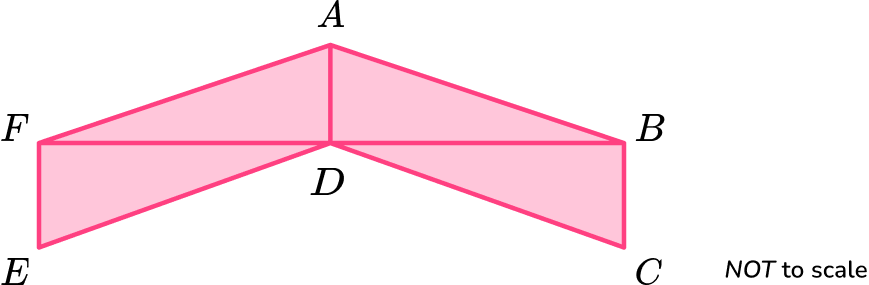100 \ cm58 \ cm120 \ cm62 \ cmAs we know that  EF, AD  and BC  are the same length and are shorter than BD  and DF , we can label each of these with the shortest side length of the triangle, 3 \ cm.

As AB, CD, DE  and AF  are all the hypotenuse of each right angle triangle, these can all be labelled as the longest side length, 13 \ cm.

This means that BD  and DF  are both 12 \ cm. We can add the dimensions of the right-angled triangles to the diagram, along with the four 90 degree angles.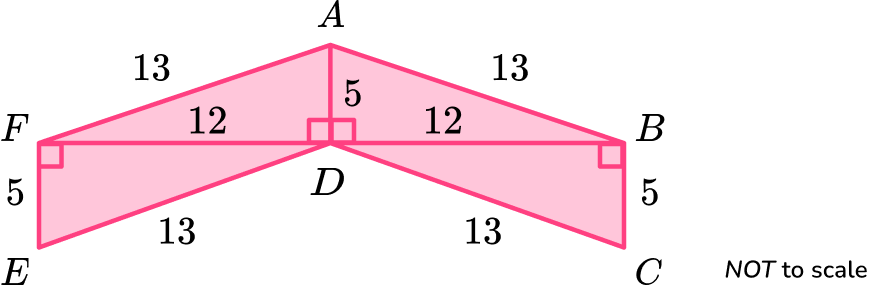The perimeter will be the sum of the sides of the shape.

13+13+5+13+13+5=62\text{ cm}

6. The sides of a right angle triangle are 7 \ cm, \ 24 \ cm, and 25 \ cm. Two of these triangles are put together to make a quadrilateral such that 2DE < CD . Calculate the perimeter of the shape.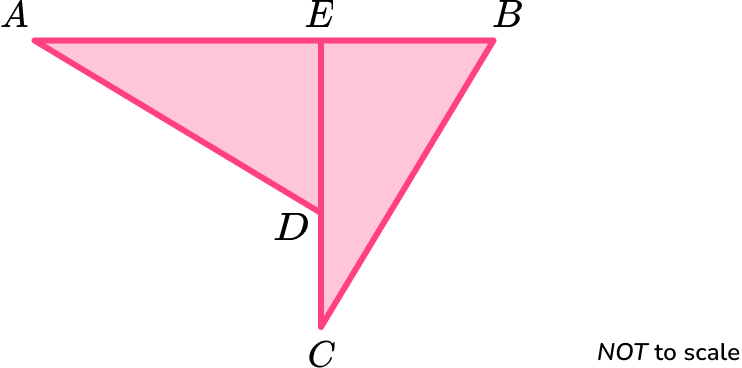98 \ cm56 \ cm112 \ cm108 \ cmWe are given that 2DE < CD . As DE  is a side length of the right-angled triangle, the length of DE  must be 7 \ cm and the length of CE  must be 24 \ cm as 2 \times 7<24-7 giving us 14<17.

The hypotenuse must be 25 \ cm as it is the longest side. We can now add the dimensions of the right-angled triangles to the diagram.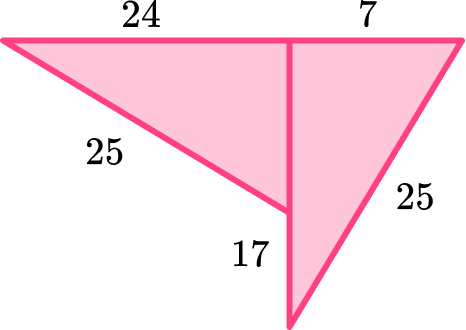We can add the lengths of the sides together to find the perimeter.

24+7+25+17+25=98\text{ cm}

The perimeter is 98\text{ cm}.

### Right angle triangle GCSE questions

1. Shape A  is a triangle.Draw on the line(s) of symmetry.

(1 mark)(1)

2. Here is a diagram.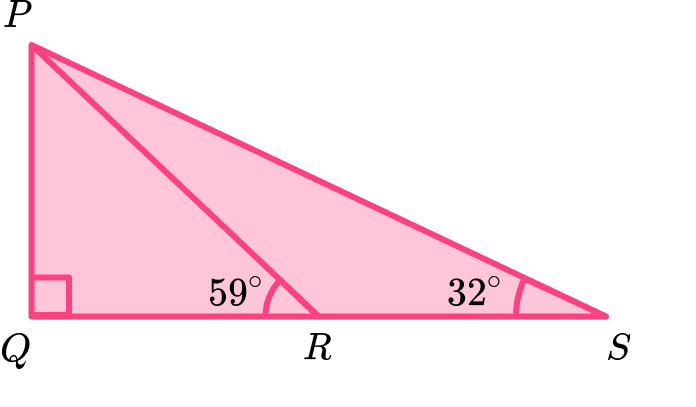PQR  and PQS  are right angle triangles.

QPR =59^{\circ} PSR =32^{\circ}

Find the size of the angle RPS .

(4 marks)

QPR  =180-90-59=31

(1)

PSR =180-90-32=58

(1)

58-31

(1)

27^{\circ}

(1)

3. An energy company is designing a new logo.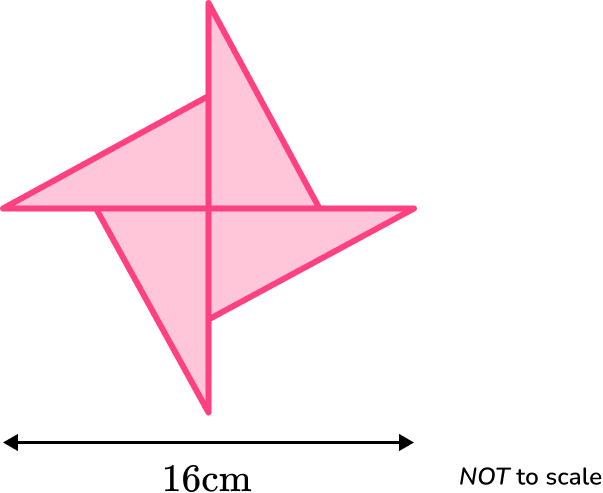It is made from four congruent triangles with sides 6 \ cm, \ 8 \ cm and 10 \ cm.

(a) Write down the order of rotational symmetry for the shape.

(b) Find the perimeter of the shape.

(4 marks)

(a) 4

(1)

(b) 8-6=2

(1)

10+2+10+2+10+2+10+2+10+2=4\times 22

(1)

88 \ cm

(1)

4. Here is a circle with a diameter AB .Bonnie says that triangle ABC  is an equilateral triangle.

Is Bonnie correct? Explain your answer clearly stating what type of triangle ABC  is.

(2 marks)

Bonnie is NOT correct. AB  is a diameter which means that angle ACB  is a right angle.

(1)

The triangle is a right angle triangle.

(1)

## Learning checklist

You have now learned how to:

• Recognise a right angle triangle
• Use the properties of a right angle triangle to solve problems

## Still stuck?

Prepare your KS4 students for maths GCSEs success with Third Space Learning. Weekly online one to one GCSE maths revision lessons delivered by expert maths tutors.

Find out more about our GCSE maths tuition programme.